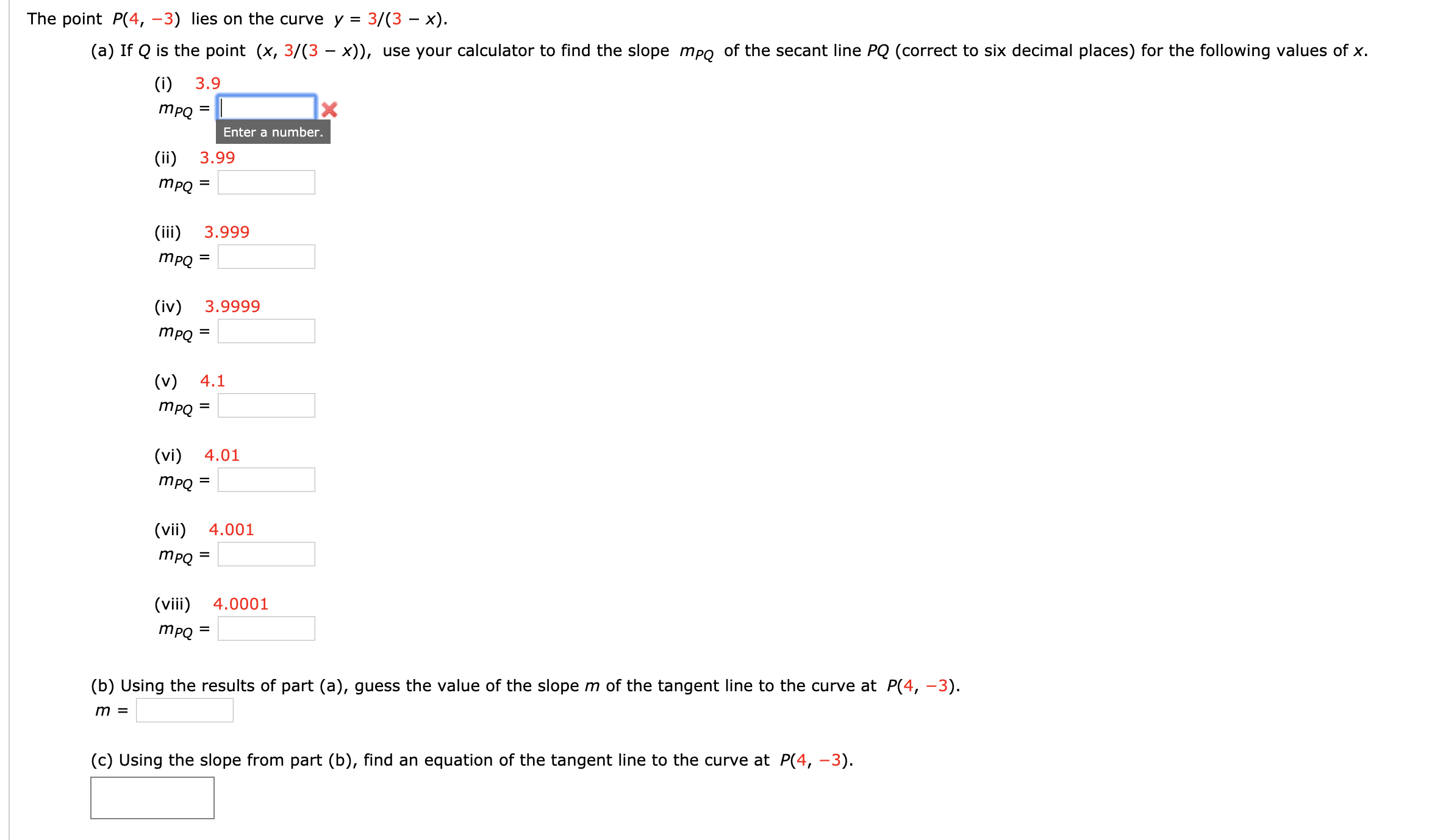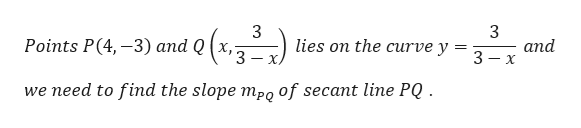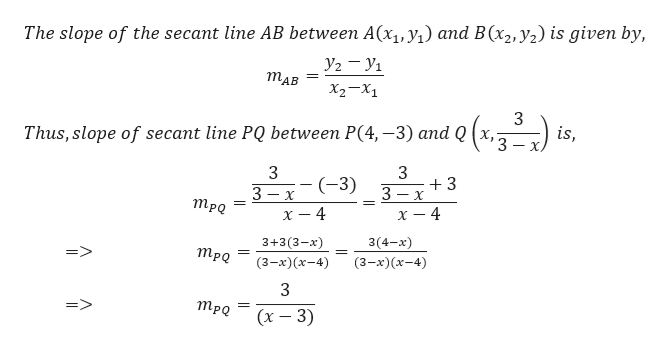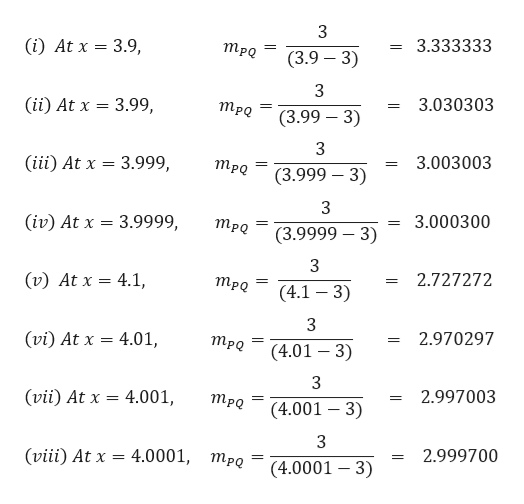# 3/(3 — х).The point P(4, -3) lies on the curve y =(a) If Q is the point (x, 3/(3 - x)), use your calculator to find the slope mpo of the secant line PQ (correct to six decimal places) for the following values of x.(i)3.9ТРОEnter a number.(ii)3.99ТРО=(iii)3.999ТРо 3(iv)3.9999mpQ=(v)4.1mpQ=(vi)4.01mро -(vii)4.001=(viii)4.0001mpQ=(b) Using the results of part (a), guess the value of the slope m of the tangent line to the curve at P(4, -3)m =(c) Using the slope from part (b), find an equation of the tangent line to the curve at P(4, -3)II

Question
156 views

I am so confused, question is in the picture.help_outlineImage Transcriptionclose3/(3 — х). The point P(4, -3) lies on the curve y = (a) If Q is the point (x, 3/(3 - x)), use your calculator to find the slope mpo of the secant line PQ (correct to six decimal places) for the following values of x. (i) 3.9 ТРО Enter a number. (ii) 3.99 ТРО = (iii) 3.999 ТРо 3 (iv) 3.9999 mpQ= (v) 4.1 mpQ = (vi) 4.01 mро - (vii) 4.001 = (viii) 4.0001 mpQ = (b) Using the results of part (a), guess the value of the slope m of the tangent line to the curve at P(4, -3) m = (c) Using the slope from part (b), find an equation of the tangent line to the curve at P(4, -3) II fullscreen
check_circle

Step 1

According to the question,help_outlineImage Transcriptionclose3 lies on the curve y 3 and 3-x Points P(4,-3) and Q (x, = 3 we need to find the slope mpo of secant line PQ fullscreen
Step 2

We know that,help_outlineImage TranscriptioncloseThe slope of the secant line AB between A(x1, y) and B(x2,y2) is given by, Тав X2-X1 3 Thus, slope of secant line PQ between P(4, -3) and Q (x,: 3 is, 3 (-3) +3 3 x 3 x x -4 - MpQ = = x - 4 3+3(3-x) 3(4-x) => Тро (3-x)(x-4) (3-x)(x-4) 3 => mpQ (х — 3) fullscreen
Step 3

We will put value of x in above expression to get...help_outlineImage Transcriptionclose(i) At x 3.9, 3.333333 mpQ = = (3.9 3) 3 (ii) At x = 3.99, 3.030303 mpQ (3.99 3) (iii) At x 3.999, 3.003003 mpQ (3.999 - 3) 3 (iv) At x 3.9999, 3.000300 mpQ (3.9999 - 3) (v) At x 4.1, 2.727272 mpQ = (4.1 3) 3 (vi) At x 4.01, 2.970297 mpQ (4.01 3) 3 (vii) At x 4.001, 2.997003 mpQ (4.001 3) 3 (viii) At x 4.0001, 2.999700 mpQ (4.0001 3) fullscreen

### Want to see the full answer?

See Solution

#### Want to see this answer and more?

Solutions are written by subject experts who are available 24/7. Questions are typically answered within 1 hour.*

See Solution
*Response times may vary by subject and question.
Tagged in

### Derivative